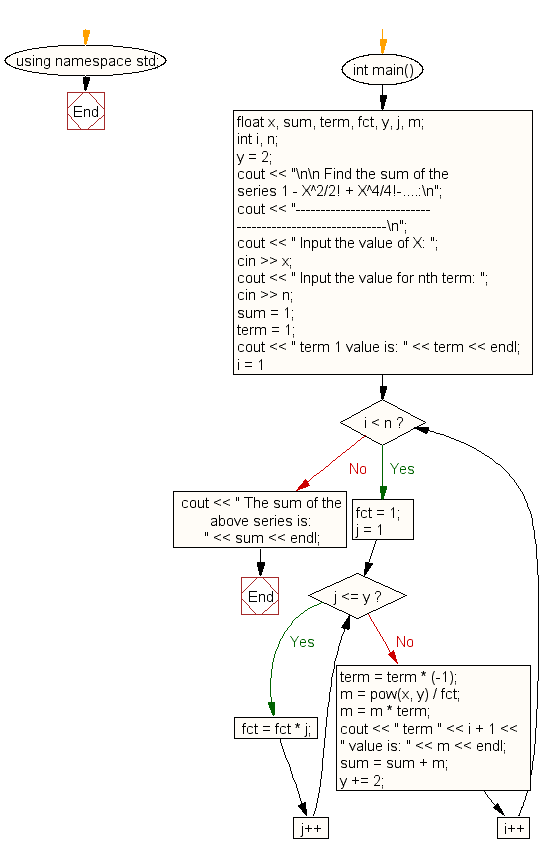﻿ C++ : Find the sum of series 1 - X^2/2! + X^4/4!-....n# C++ Exercises: Find the sum of series 1 - X^2/2! + X^4/4!-.... upto nth term

## C++ For Loop: Exercise-14 with Solution

Write a program in C++ to find the sum of series 1 - X^2/2! + X^4/4!-.... upto nth term.

Sample Solution :-

C++ Code :

``````#include <iostream>
#include <math.h>
using namespace std;

int main()
{
float x, sum, term, fct, y, j, m;
int i, n;
y = 2;

cout << "\n\n Find the sum of the series 1 - X^2/2! + X^4/4!-....:\n";
cout << "---------------------------------------------------------\n";
cout << " Input the value of X: ";
cin >> x;
cout << " Input the value for nth term: ";
cin >> n;
sum = 1;
term = 1;
cout << " term 1 value is: " << term << endl;
for (i = 1; i < n; i++)
{
fct = 1;
for (j = 1; j <= y; j++)
{
fct = fct * j;
}
term = term * (-1);
m = pow(x, y) / fct;
m = m * term;
cout << " term " << i + 1 << " value is: " << m << endl;
sum = sum + m;
y += 2;
}
cout << " The sum of the above series is: " << sum << endl;
}
``````

Sample Output:

``` Find the sum of the series 1 - X^2/2! + X^4/4!-....:
---------------------------------------------------------
Input the value of X: 3
Input the value for nth term: 4
term 1 value is: 1
term 2 value is: -4.5
term 3 value is: 3.375
term 4 value is: -1.0125
The sum of the above series is: -1.1375
```

Flowchart:C++ Code Editor:

Contribute your code and comments through Disqus.

What is the difficulty level of this exercise?

﻿

## C++ Programming: Tips of the Day

What is a smart pointer and when should I use one?

This answer is rather old, and so describes what was 'good' at the time, which was smart pointers provided by the Boost library. Since C++11, the standard library has provided sufficient smart pointers types, and so you should favour the use of std::unique_ptr, std::shared_ptr and std::weak_ptr.

There was also std::auto_ptr. It was very much like a scoped pointer, except that it also had the "special" dangerous ability to be copied - which also unexpectedly transfers ownership.

It was deprecated in C++11 and removed in C++17, so you shouldn't use it.

```std::auto_ptr<MyObject> p1 (new MyObject());
std::auto_ptr<MyObject> p2 = p1; // Copy and transfer ownership.
// p1 gets set to empty!
p2->DoSomething(); // Works.
p1->DoSomething(); // Oh oh. Hopefully raises some NULL pointer exception.
```

Ref : https://bit.ly/3mc9GHE